Home > CCA2 > Chapter 12 > Lesson 12.2.2 > Problem12-111

12-111.
1.Without using a calculator, solve each equation below in the domain 0 ≤ x < 2π. Homework Help ✎

1. sin (x) = −1

2. 2 cos (x) − 1 = 0

3. tan (x) = 1

4. 2 sin (x) = 4 sin (x) + 1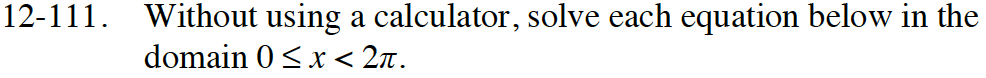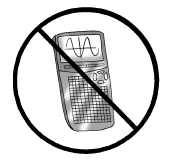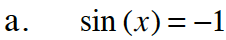Reference the unit circle to help solve this problem.
For which angle(s) in the circle does sin(x) = −1.

$x=\frac{3\pi}{2}$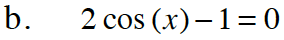Solve the equation for cos(x).

$\cos(x) = \frac{1}{2}$

For which angle(s) in the unit circle does cos(x) = 1/2?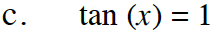Refer to part (a).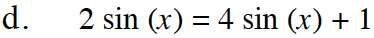How would you solve 2m = 4m + 1?
In this case m = sin(x).

$x=\frac{7\pi}{6},\frac{11\pi}{6}$